## Friday, December 29, 2006

### Van Roomen's Problem

Adriaan Van Roomen was a professor of mathematics and medicine in Louvain who had developed a strong reputation as one of the top mathematicians of his age. In 1593, he published a survey of the most important living mathematicians. In this work, he proposed a math problem that he dared any of these top mathematicians to solve:

(45)x - (3,795)x3 + (95,634)x5 - (1,138,500)x7 + (7,811,375)x9 - (34,512,075)x11 + (105,306,075)x13 - (232,676,280)x15 + (384,942,375)x17 - (488,494,125)x19 + (483,841,800)x21 - (378,658,800)x23 + (236,030,652)x25 - (117,679,100)x27 + (46,955,700)x29 - (14,945,040)x31 + (3,764,565)x33 - (740,259)x35 + (111,150)x37 - (12,300)x39 + (945)x41 - (45)x43 + x45 = A

where: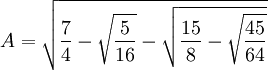To show that Van Roomen had a solid understanding of this monstrous equation, he observed the following:

(a) If:Then: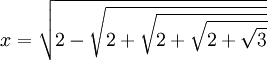(b) If:Then:(c) If: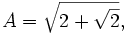Then:This problem was mentioned in a meeting between the Dutch ambassador and the King Henry IV of France. The Dutch ambassador had noted that not a single French mathematician had been listed in van Roomen's survey of the great mathematicians.

King Henry IV presented the problem to François Viète who was able to solve it. Viète's solution is historically significant because he not only demonstrated how trigonometry can be used to solve algebraic equations, he also showed that this problem possessed 45 different solutions. This result was important in helping to establish the Fundamental Theorem of Algebra.

How did Viète solve it? I will show his solution in my next blog.

References
• Eli Maor, Trigonometric Delights, Princeton Paperbacks, 1998
• Jean-Pierre Tignol, Galois' Theory of Algebraic Equations, World Scientific, 2001

## Sunday, December 10, 2006

### François Viète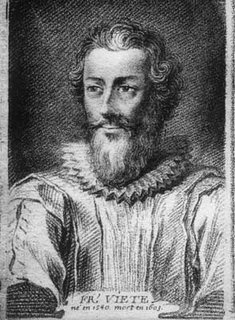François Viète was born in 1540 in France; he was a successful politician who also made very important contributions to algebra. He is credited for example with introducing letters to represent known and unknown values in mathematical equations. His writings were also important in establishing the symbol (+) as representing addition and (-) as representing subtraction.

His family was well connected; his mother, for example, was the first cousin of the president of Parliament in Paris. Viète was able to take advantage of these connections through out his life. He attended the University of Poitiers and in 1560, he graduated with a law degree.

Although a lawyer by profession, he took a strong interest in math and science and published his first mathematical paper in 1571. He was especially interested in the works of Pappus and Diophantus.

Viète was a Huguenot and he lived in Paris during the St. Bartholomew's Day Massacre where many thousands of protestant Hugeuenots were killed.

In 1573, he became a councillor at Rennes where he remained until 1580. At that time, he was appointed by King Henry III to be a royal privy councillor in Paris. In 1584, King Henry III's younger brother became ill and died; this meant that Henry of Navarre, a protestant, became heir to the throne. In the struggle that erupted, Viète, a protestant, was kicked out of office.

Viète left Paris and headed to the small town of Beauvoir-sur-Mer. He spent five years there where he was able to devote time to studying mathematics. It was during this time that he did most of his most imporant work relating to cubic equations and mathematical notation.

Viète believed that the Greeks had not revealed all their mathematical insights. He believed that they had secret methods which he hoped to rediscover. In this way, he introduced what would later become variables and coefficients. In Viète's view, he had restored the hidden mathematical methods of the Greeks.

In 1589, he was called back to parliament in Tours. This happened just before Henry III was assassinated on August 1, 1589. He stayed on as the protestant Henry of Navarre became King Henry IV.

In 1590, a coded letter to King Philip II of Spain was intercepted by the French. Viète, at this time, had a very strong reputation in mathematics and he was given the task of deciphering the note. This he accomplished in March of 1590. The MacTutor web site quotes a historical text:
... when Philip, assuming that the cipher could not be broken, discovered that the French were aware of his military plans, he complained to the Pope that black magic was being employed against his country.
When in 1592, King Henry IV converted to Catholicism. Viète did the same.

In 1593, there was a challenge made to all mathematicians by Adriaan van Roumen, a professor of math at Louvain. Roumen asked for a solution to an equation which had 45 terms. Viète was able to solve this problem. In fact, Roumen asked for one solution. Viète was able to demonstrate that there were 23 positive solutions.

Viète was very wealthy. He published all of his writings himself and sent them out to scholars throughout Europe. In all of his writings, he only focuses on positive values. Like many of the mathematicians before him, he did not include consideration of negative numbers in his writings.

He was a member of the Royal Privy Council until his death in February, 1603.

References

## Saturday, December 09, 2006

### Proof of the Solution to the General Quartic Equation

In today's blog, I provide the proof for the solution of the general quartic equation. If you would like to see how Lodovico Ferrari found his solution, see here.

The content in today's blog is taken from Jean-Pierre Tignol's Galois' Theory of Algebraic Equations.

Lemma 1: Depressed Quartic Equation

If:

x4 + bx3 + cx2 + dx + e = 0

Then there exists y,p,q,r such that:

y4 + py2 + qy + r = 0

where:

y = x + (b/4)

p = c - 6(b/4)2

q = d - (b/2)c + (b/2)3

r = e - (b/4)d + (b/4)2c - 3(b/4)4

Proof:

(1) Let y = x + (b/4) so that x = y - (b/4)

(2) We note that (see here for details on the Binomial Theorem if needed):

(u - v)2 = u2 - 2uv + v2

(u - v)3 = u3 - 3u2v + 3uv2 -v3

(u - v)4 = u4 - 4u3v + 6u2v2 -4uv3 + v4

(3) This then gives us:

(y - [b/4])4 = y4 - by3 + (3/8)b2y2 -b3y/16 + (b/4)4

b(y - [b/4])3 = by3 - (3/4)b2y2 + (3/16)b3y - b4/64

c(y - [b/4])2 = cy2 - bcy/2 + c(b/4)2

d(y - [b/4]) = dy - bd/4

(4) Putting this all together gives us:

x4 + bx3 + cx2 + dx + e =

= y4 + (3/8)b2y2 -b3y/16 + (b/4)4 - (3/4)b2y2 + (3/16)b3y - b4/64 + cy2 - bcy/2 + c(b/4)2 + dy - bd/4 +e =

= y4 + [c-(3/8)b2]y2 + [ (1/8)b3 -bc/2 + d]y + [e - bd/4 + c(b/4)2 - 3b4/256 ]

QED

Lemma 2: Solution for y4 + py2 + r = 0

If y4 + py2 + r = 0

Then:

y = ± √ (1/2)[-p ± √p2 - 4r

Proof:

(1) y4 + py2 + r = 0

(2) (y2)2 + p(y2) + r = 0

(3) Using the quadratic equation (see here):

y2 = (1/2)[-p ± √p2 - 4r

(4) Solving for y2, we get:

y = ± √ (1/2)[-p ± √p2 - 4r

QED

Lemma 3:

If:

y4 + py2 + qy + r = 0 and q ≠ 0

Then there exists u ≠ 0 such that:

(y2 + p/2 + u)2 = [√2uy - q/(2√2u)]2

Proof:

(1) y4 + py2 + qy + r = 0

(2) y4 + py2 + (p/2)2 = -qy -r + (p/2)2

(3) (y2 + p/2)2 = -qy -r + (p/2)2

(4) Let u be the solution of the following equation:

8u3 + 8pu2 + (2p2 -8r)u - q2 = 0.

We know that this solution exists from the general cubic equation (see here)

(5) We know that u ≠ 0 since if u = 0, then q = 0 but q ≠ 0.

(6) So, we can rearrange our equation in step #4 to:

8u3 + 8pu2 + (2p2 -8r)u = q2

And after dividing both sides by 8u, we get:

u2 + pu + (p/2)2 - r = q2/8u.

(7) Now, we know that:

(y2 + p/2 + u)2 = -qy -r + (p/2)2 + 2uy2 + pu + u2

(8) So, using step #6, we have:

-qy -r + (p/2)2 + 2uy2 + pu + u2 =

= -qy + 2uy2 + q2/8u =

= [√2uy - q/(2√2u)]2

QED

Thereom: General Quartic Equation

If:

ax4 + bx3 + cx2 + dx + e = 0

p = (c/a) - 6([b/a]/4)2

q = (d/a) - ([b/a]/2)c + ([b/a]/2)3

r = (e/a) - ([b/a]/4)d + ([b/a]/4)2c - 3([b/a]/4)4

s = ([b/a]/4)

u = the solution to the cubic equation: 8u3 + 8pu2 + (2p2 -8r)u - q2 = 0.

Then:

if q = 0,

x = ± √ (1/2)[-p ± √p2 - 4r - s

if q ≠ 0, then:

x = ± (1/2) [√2uy + √2u - 2p - 4u + 2q/(√2u)] - s

or

x = ± (1/2) [-√2uy + √2u - 2p - 4u - 2q/(√2u)] - s

Proof:

(1) ax4 + bx3 + cx2 + dx + e = 0

(2) If we divide all sides by a, we get:

x4 + (b/a)x3 + (c/a)x2 + (d/a)x + (e/a) = 0

(3) Using Lemma 1 above, we get:

y4 + py2 + qy + r = 0

where:

y = x + ([b/a]/4)

p = (c/a) - 6([b/a]/4)2

q = (d/a) - ([b/a]/2)c + ([b/a]/2)3

r = (e/a) - ([b/a]/4)d + ([b/a]/4)2c - 3([b/a]/4)4

(4) If q=0, then using Lemma 2 above, we have:

y = ± √ (1/2)[-p ± √p2 - 4r

(5) If q ≠ 0, then using Lemma 3 above, we have:

(y2 + p/2 + u)2 = [√2uy - q/(2√2u)]2

where

u is the solution to:

8u3 + 8pu2 + (2p2 -8r)u - q2 = 0.

We can find this solution using the cubic equation (see here).

(6) This then gives us:

y2 + p/2 + u = ± √2uy - q/(2√2u).

(7) This then gives us four solutions:

(a) Case I: y2 + p/2 + u = + √2uy - q/(2√2u).

In this case:

y2 - √2uy + [p/2 + u + q/(2√2u)] = 0

y = ± (1/2) [√2uy + √2u - 2p - 4u - 2q/(√2u)]

(b) Case I: y2 + p/2 + u = - √2uy + q/(2√2u).

In this case:

y2 + √2uy + [p/2 + u - q/(2√2u)] = 0

y = ± (1/2) [-√2uy + √2u - 2p - 4u + 2q/(√2u)]

(8) Since x = y - ([b/a]/4), we have the following solutions:

(a) If q=0, then:

x = ± √ (1/2)[-p ± √p2 - 4r - ([b/a]/4)

(b) If q ≠ 0, then:

x = ± (1/2) [√2uy + √2u - 2p - 4u - 2q/(√2u)] - ([b/a]/4)

or

x = ± (1/2) [-√2uy + √2u - 2p - 4u + 2q/(√2u)] - ([b/a]/4)

QED

References

## Saturday, December 02, 2006

### Ferrari's Solution to the quartic equation

In today's blog, I go through Lodovico Ferrari's solution of the general quartic equation. In my next blog, I will present a proof of Ferrari's method.

The content in today's blog is taken from Jean-Pierre Tignol's Galois' Theory of Algebraic Equations.

(1) Ferrari started out with a quadratic equation of the following form:

x4 + bx3 + cx2 + dx + e = 0

(2) Then, he borrowed a method from Girolamo Cardano (see here) and set y = x + (b/4) which means x = y - (b/4).

(3) Substituting x = y - (b/4) gives us:

(y - [b/4])4 + b(y - [b/4])3 + c(y - [b/4])2 + d(y - [b/4]]) + e = 0

Working this through (see details here), gives us:

y4 + py2 + qy + r = 0

where:

p = c - 6(b/4)2

q = d - (b/2)c + (b/2)3

r = e - (b/4)c + (b/4)2c - 3(b/4)4

(4) Now if we move the q and r term over we get:

y4 + py2 = -qy -r

This helps us because if we add (p/2)2 to both sides, we can complete the square to get:

(y2 + p/2)2 = -qy -r + (p/2)2

(5) Now, if we add a value u to our square, we get the following:

(y2 + p/2 + u)2 = -qy -r + (p/2)2 + 2uy2 + pu + u2

This may not appear to help us but it gives us a possibility for completing the square on the other side.

(6) For example, if we could find a value u such that:

-qy -r + (p/2)2 + 2uy2 + pu + u2 = ([√2uy - q/(2√2u)]2

Then we have found a solution (see step #11 if this point is not clear)

(7) Now:

([√2uy - q/(2√2u)]2 = 2u2y2 - 2*(√2uy)(q/(2√2u)) + q2/(8u) =

= 2u2y2 - q/u + q2/(8u)

(8) So this equation works only if:

-qy -r + (p/2)2 + 2uy2 + pu + u2 = 2uy2 - qy + q2/(8u)

That is if:

-r + (p/2)2 + pu + u2 = q2/(8u)

(9) But if we multiply 8u to all sides and then subtract by q2, we get:

8u3 + 8pu2 + (2p2 - 8r)u - q2 = 0

(10) This is the general cubic equation which we can solve using Cardano's formula so we know that u can take on this value.

(11) This then gives us:

(y2 + p/2 + u)2 = ([√2uy - q/(2√2u)]2.

(12) This can we solve using the quadratic equation. The four solutions can be found from:

(y2 + p/2 + u)2 = ± [√2uy - q/(2√2u)]

I should probably note that we assumed that u ≠ 0. I will talk about the situation were u = 0 in my next blog where I provide a proof for Ferrari's solution.

References

## Friday, December 01, 2006

### Bombelli and the invention of complex numbers

Rafael Bombelli was the first to propose the idea of complex numbers.

Bombelli wrote about imaginary numbers in his very influential book Algebra which was published in 1572.

Bombelli based his argument for their usefulness on Girolamo Cardano's solution for the cubic equation. Consider the following equation:

x3 = 15x + 4.

This is an example of the depressed cubic so we can use del Ferro/Tartaglia solution (see here):

b=-15
c=4

with:This then gives us:So that: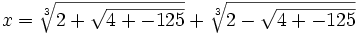which simplies to: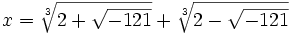Cardano had considered such forms "irreducible" and had ignored them. Bombelli noticed that this wasn't quite right. For example, x3 = 15x + 4 is solved when x=4.

Bombelli proposed what he called a "wild thought". What if the term -1 could be used mathematically so that the above value could be reduced to a number of the form: a+b√-1.

Bombelli proposed mathematical rules for allowing such numbers:

b1-1 + b2-1 = (b1 + b2)√-1

(b1-1)*(b2-1) = (-1)b1b2

and finally if:

a + bi = c + di, then a=c and b=d.

Then, using these assumptions, here's what he found:

(1) Assume that:

( a + b√-1)3 = 2 + √-121

(2) We note that (m + n)3 = m3 + n3 + 3mn(m + n) since:

(m + n)3 = m3 + 3m2n + 3mn2 + n3 = m3 + n3 + 3mn(m + n)

(3) Let m = a, n=b√-1 which gives us:

( a + b√-1)3 = a3 + b3(-1)√-1 + 3(a)(b√-1)(a + b√-1) =

=a3 - b3-1 + 3a2(b√-1) + 3ab2(-1) =

= a(a2 -3b2) + b(3a2 - b2)√-1.

(4) Now solving for ( a + b√-1)3 = 2 + √-121 gives us:

a(a2 -3b2) = 2

b(3a2 - b2) = 11

(5) Since 2,11 are primes, we know that in both cases, one of the two factors must be 1.

If we assume that a=2,b=1, then we see that:

22 - 3(12) = 1
3*22 - 12 = 11

(6) So that:

(2 + √-1)3 = 2 + √-121.

(7) And using the same reasoning, we see that:

(2 - √-1)3 = 2 - √-121.

(8) If we assume that his reasoning is sound, we now find that:reduces to:

x = (2 + √-1) + (2 - √-1) = 4

In this way, the theory of complex numbers was first presented by Bombelli and was eventually accepted by mathematicians.

References

## Monday, November 27, 2006

### Rafael Bombelli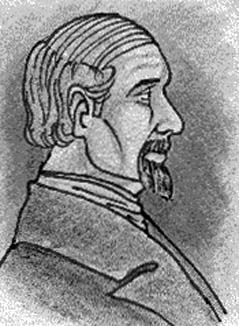Rafael Bombelli was born in 1526 in Bologna. His father, Antonio Mazzoli, changed his name to Bombelli in order to avoid the reputation of the Mazzoli family. The Mazzoli family had once been very powerful and influential in Bologna. This all changed when Pope Julius II came to power. In 1506, the ruling family, the Bentivoglios, were exiled. In 1508, there was an effort to take back Bologna which failed. Mazzoli's grandfather was among those captured and executed. Later, Antonio Bombelli, was able to make his way back to Bologna as a wool merchant.

Rafael was the eldest of six children of Antonio Bombelli. He did not go to the university but learned mathematics as an engineer. He took a deeper interest in mathematics and managed to read many of important math works of his day.

At this time, there was great publicity regarding the controversy around the solution of the cubic equation between Girolamo Cardano and Nicolo Fontana Tartaglia.
It is clear that Bombelli came to read Cardano's Ars Magna and also that he closed followed the debate between Tartaglia and Lodovico Ferrari in Milan in 1548.

We know that in 1549, Bombelli was tasked by Alessandro Rufini to work on a major engineering project to reclaim marshlands. He continued to work on this project unil 1555 when the work there was put on hold.

It was at this time, during the interruption of work, that Bombelli began work on a math book on algebra. He believed that the only work which delved deeply into the subject, Cardano's work, was too difficult and subtle for a majority of mathematicians. He sought to provide a clearer and more accessible version.

In 1560, Bombelli resumed the major engineering project. The project was deemed a giant success and afterwards, Bombelli found himself with a reputation as a very talented hydraulic engineer. He was now tasked to do other very important projects.

One time, when he was traveling in Rome, he learned about Diophantus's Arithmetica. He had not yet finished his intended book on algebra so his reading of Diophantus had a great impact on his math project. Bombelli then published the first three parts of his algebra in 1572.

Bombelli's Algebra is one of the most important and influential works ever published. It is a thorough and in depth analysis of the state of algebra. In it, he introduces the idea of complex numbers for the first time. Others had noticed the idea of negative numbers and the possibility of negative roots that appear in Cardano's cubic equation but it was Bombelli who first defended them as worthy of study in themselves.

Bombelli proposed a mathematical notation that was a significant advancement at the time.He also proposes rules for addition, subtraction, and multiplication of complex numbers. Most importantly, he showed how reasoning about complex numbers using Cardano's cubic equation could lead to real values. I will go into more detail about this in my next blog.

Bombelli had intended to publish two more parts but he did not finish them. He died in 1572. The last two parts were later found and published in 1929. Today, Bombelli is considered the inventor of complex numbers.

References

## Saturday, November 25, 2006

### Solution to the general cubic equation

Girolamo Cardano was able to find a general solution to the cubic equation based on Nicolo Fontana Tartaglia's solution to the depressed cubic equation.

For an equation of the form x3 + bx2 + cx + d = 0, Cardano did this by setting x = y - b/3.

The solution is complicated. Still, this solution was a very important step forward past the mathematics of Ancient Greece. Additionally, it would lead eventually to the acceptance of imaginary numbers and the establishment of group theory.

Lemma 1: Cardano's simplification of general cubic equation

If:

x3 + bx2 + cx + d = 0

Then there exists y,p,q such that:

y3 + py + q = 0

Proof:

(1) x3 + bx2 + cx + d = 0

(2) Let y = x + b/3

(3) Then, x = y - b/3

(4) x3 = (y - b/3)3 = y3 - 3by2/3 + 3b2y/9 - b3/27 =

= y3 - by2 + b2y/3 - b3/27

(5) bx2 = b(y - b/3)2 = by2 - 2b2y/3 + b3/9

(6) x3 + bx2 = y3 - b2y/3 + 2b3/27

(7) x3 + bx2 + cx + d =

= y3 - b2y/3 + 2b3/27 + c(y - b/3) + d =

= y3 + (c - b2/3)y + (2b3/27 - bc/3 + d)

(8) Let:

p = c - b2/3

q = 2b3/27 - bc/3 + d

QED

Theorem: Solution to General Cubic Equation

If:

ax3 + bx2 + cx + d = 0

Then: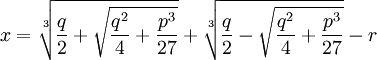where: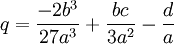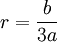Proof:

(1) ax3 + bx2 + cx + d = 0

(2) If we divide all sides by a, we get:

x3 + (b/a)x2 + (c/a)x + (d/a) = 0

(3) Then using Lemma 1 above, if we set:

y = x - (b/a)/3

p = (c/a) - (b/a)2/3

q = -2(b/a)3/27 + (b/a)(c/a)/3 - (d/a)

Then we have:

y3 + py - q = 0

or:

y3 + py = q

(4) Now, using the solution for the depressed cubic (see Theorem, here),

we have: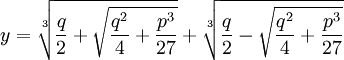(5) Finally, adjusting for step #3, if we let:

r = (b/a)/3

Then we have:QED

### Proof of Solution to Depressed Cubic

In today's blog, I will show a proof of the solution to the depressed cubic equation. Using Girolamo Cardano's trick (next blog), it is possible to use the solution to the depressed cubic equation to solve the general cubic equation.

Lemma 1: x3 = bx + c → ∃ u,v such that: b=3uv, c=u3 + v3, x = u + v

Proof:

(1) Let x3 = bx + c.

(2) Let x = u + v

(3) (u + v)3 = u3 + 3u2v + 3uv2 + v3 = 3uv(u + v) + (u3 + v3)

(4) 3uv(u + v) + (u3 + v3) = b(u + v) + c

(5) So that b = 3uv and c = u3 + v3

QED

Lemma 2: if b=3uv and c=u3 + v3, then:

we can assume:

u3 = c/2 + √(c/2)2 - (b/3)3

v3 = c/2 - √(c/2)2 - (b/3)3

Proof:

(1) b=3uv → v = b/3u

(2) u3 + v3 = u3 + (b/3u)3 =

= u3 + b3/(27u3) = c

(3) So that we have:

u3 + b3/(27u3) - c = 0

(4) Multiplying both sides by u3 gives us:

u6 + b3/27 - cu3 = 0

(5) Using the solution for quadratic equations (see here) gives us:

u3 = (c/2) ± √(c/2)2 - (b/3)3

(6) Since u3 + v3 = c, we can assume that:

u3 = (c/2) + √(c/2)2 - (b/3)3

v3 = (c/2) - √(c/2)2 - (b/3)3

QED

Theorem: Solution of the Depressed Cubic

if:

x3 + bx = c

then:Proof:

(1) x3 + bx = c

(2) So that we have:

x3 = (-b)x + c

(3) Using Lemma 1 above, we see that there exists u,v such that:

x = u + v

(-b) = 3uv

c = u3 + v3

(4) Using Lemma 2 above, we see that:

u3 = (c/2) + √(c/2)2 - (-b/3)3 = (c/2) + √(c/2)2 + (b/3)3

v3 = (c/2) - √(c/2)2 - (-b/3)3 = (c/2) - √(c/2)2 + (b/3)3

(5) Since x = u+v, we have:QED

## Friday, November 24, 2006

### Depressed Cubic

Scipione del Ferro's great discovery which eventually led to the general solution of the cubic equation was the solution of the depressed cubic equation:

x3 + bx = c

In today's blog, I will show how del Ferro solved this equation. The content is taken from Paul J. Nahin's The Story of √-1

(1) Let x = u+v

(2) Then:

x3 + bx = (u + v)3 + b(u + v) =

= u3 + 3u2v + 3uv2 + v3 + b(u + v) =

= u3 + v3 + (3uv + b)(u + v) = c

(3) Now, if:

(3uv + b) = 0

then:

u3 + v3 = c

(4) Now,

(3uv + b) = 0 → 3uv = -b

and further:

v = -b/(3u)

(5) So if:

u3 + v3 = c, then:

u3 + [-b/(3u)]3 - c =

u3 + (-b3)/(27u3) - c =

u6 + (-b3/27 - cu3 = 0

(6) But this is an example of a quadratic equation since if:

z = u3, then we have:

u6 + (-b3/27) - cu3 =

= z2 - cz -(b3/27)

(7) Using the solution for the quadratic equation (see here), we have:

z = (c/2) ± √c2 - 4(-b3/27)/2 =

=(c/2) ± √c2/4 + b3/27 = u3

(8) Considering only the positive form, we have: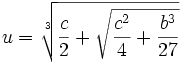(9) Since v3 = c - u3, we have:

v3 = c - (c/2 + √c2/4 + b3/27 =

= c/2 - √c2/4 + b3/27

(10) This then gives us: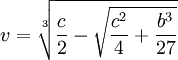(11) Since x = u + v, this gives us that:(12) Since (-1)3 = (-1)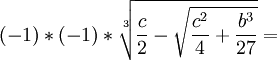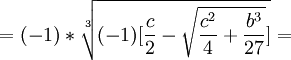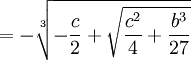(13) This tells us that we can restate (11) as the following: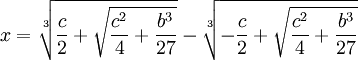In another blog, I present a proof that this really is the solution of the depressed cubic.

References

## Wednesday, November 22, 2006

### Nicolo Fontana Tartaglia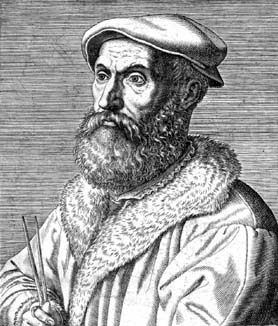Nicolo Fontana "Tartaglia" was born around 1500 in Venice. His father was a "mail rider", a person who would ride from town to town, to make deliveries. When Tartaglia was six years old, his father was murdered during one of these trips.

When Tartaglia was 12, the French took over his town of Brescia. There had been fierce fighting and in retaliation, there was a great slaughter of the Brescian townfolks. It is said that 46,000 inhabitants were killed in the fighting and the revenge killings. Tartaglia, his mother, and his sister hid away in a cathedral. In the course of the fighting, Tartaglia was attacked and left for dead.

Somehow, he survived but for the rest of his life, he bore terrible scars near his jaw and had difficulty speaking. It was in this way that he got his nickname "Tartaglia" which means "the stammerer". As an adult, he would always wear a beard in order to hide the scars.

Tartaglia took an interest in mathematics and demonstrated strong ability which earned him the patronage of Ludovico Balbisonio who paid for him to attend the University of Padua. By 1516, he had moved to Verona where he made his living by lecturing on mathematics.

In 1534, Tartaglia was working as a math teacher in Venice. While this was still a low paying position, he began to develop a reputation by participating in public mathematical challenges.

In 1535, there was a famous math contest between Tartaglia and Fior. Fior was a student of Scipione del Ferro who had already solved the depressed cubic equation and had given the solution to Fior before he died. Tartaglia had by this time found a solution to one form of the cubic equation which was not directly solvable by the depressed cubic equation. Both Tartaglia and Fior submitted 30 problems that the other had to solve. Tartaglia could tell that Fior had a solution to the depressed cubic so he worked hard to find it. On February 13, 1535, Tartaglia solved the depressed cubic. Tartaglia easily solved all 30 of Fior's problems and won the contest.

Girolamo Cardano heard about Tartaglia's solution of the depressed cubic. Cardano, by this time, had a reputation as a brilliant and well-connected mathematician and physician. He approached Tartaglia with the hope of including Tartaglia's result in a book he was working on. Tartaglia declined.

Cardano promised to provide a letter of introduction to a very influential patron in Milan and invited Tartaglia to his home. Tartaglia, who still held his teacher's job, accepted Cardano's invitation to visit. While there, Cardano was able to convince Tartaglia to reveal his method for solving the depressed cubic. Cardano promised that he would not publish the solution without getting permission from Tartaglia.

Using Tartaglia's method, Cardano was able to solve the general cubic equation and his assistant Lodovico Ferrari was able to solve the general quartic equation. Tartaglia refused to give Cardano permission to publish the method. It is probable that Tartaglia believed that he could use his method to continue to win math challenges.

When Cardano learned that Scipione del Ferro was the first to solve the depressed cubic, he decided to go ahead and publish his book Ars Magna even without Tartaglia's permission. In the book, he gives Tartaglia and del Ferro credit for the solving the depressed cubic. He then shows how the general cubic equation can be reduced to a form of the depressed cubic.

This publishing of Cardano's book infuriated Tartaglia who proceeded to publish his own book called New Problems and Inventions. In it, he attacked Cardano. Despite this, Cardano's book was a bestseller and established Cardano as the leading mathematician of his age.

Tartaglia challenged Cardano to a public math debate but Cardano refused. Cardano's assistant Ferrari offered to take up the challenge in Cardano's place. At this time, Ferrari was a relatively unknown mathematician and Tartaglia had little to gain from the challenge. On the other hand, if he had succeeded in challenging Cardano, because of Cardano's reputation, the debate could have helped his mathematical standing.

Tartaglia was offered a very lucrative post that he could get if he defeated Ferrari in the challenge. The math debate between Tartaglia and Ferrari occurred on August 10, 1548. Ferrari had clearly mastered the general cubic and quartic equations and it was very clear that Tartaglia would not be able to answer all of Ferrari's problems. Rather than admit this, Tartaglia left before the challenge completed.

Having lost the challenge, Tartaglia found that his existing position was threatened. In this way, Tartaglia saw his reputation collapse. It is said that for the rest of his life, he harbored resentment against Cardano. It is also said that he died in poverty. Tartaglia died on December 13, 1557.

References

### Scipione del Ferro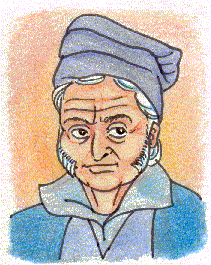Scipione del Ferro was the first mathematician to find a solution to the depressed cubic equation which is the equation of the form:

ax3 + bx = c

Gerolamo Cardano would later show how the solution to the depressed cubic equation leads to the solutions of the general cubic equation.

Del Ferro was born on February 6, 1465 in Bologna, Italy. His father was a papermaker which was a profession that was growing in importance due the invention of the printing with moveable types (this was invented by Johannes Gutenberg in 1447). Not much is known about del Ferro's early life but it is believed that he attended University of Bologna which had been founded in the eleventh century.

In 1496, del Ferro became a lecturer in mathematics at the University of Bologna. If del Ferro wrote any books, none of them survive. It is believed that he was very secretive about his discoveries and communicated them only with close friends and selected students. He kept his discoveries in a notebook. This notebook would later become an important record in establishing that del Ferro had indeed discovered the solution to the depressed cubic equation.

There is a story often told that del Ferro kept his solution to the depressed cubic equation secret until on his deathbed, he revealed it. According to the MacTutor biography, this story is false. There are numerous people who had knowledge of del Ferro's solution.

Del Ferro is believed to have died on November 5, 1526. On his death, his notebook was given to his son-in-law Hannibal Nave. It was discovery of this notebook that motivated Cardano to release his famous math book Ars Magna. Cardano had promised Tartaglia that he would not reveal the method but he felt that del Ferro's earlier discovery of the method excused him from this promise.

Today, with the notebook lost, it is very difficult to assess the mathematical contributions of del Ferro. There is no doubt that he was the first to solve a very difficult problem which would later give birth to imaginary numbers and group theory.

Cardano would later write (quoted from MacTutor biography on del Ferro):

Scipione Ferro of Bologna, almost thirty years ago, discovered the solution of the cube and things equal to a number [which in today's notation is the case x3+ mx = n], a really beautiful and admirable accomplishment. In distinction this discovery surpasses all mortal ingenuity, and all human subtlety. It is truly a gift from heaven, although at the same time a proof of the power of reason, and so illustrious that whoever attains it may believe himself capable of solving any problem.

References